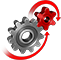# WolframSystemModeler

## Hydraulic Motor

Hydraulic motors, or rotary actuators, convert fluid power to rotary motion. They are rated according to displacement, torque capability, speed, and maximum pressure limitations. Here, we study how two of these variables relate by looking at the effect of displacement on motor speed.

### The Model

For our analysis, we use the built-in hydraulic motor and pump models in the Wolfram Hydraulic library. The pump model is set to have a fixed displacement and to operate at a constant speed. The displacement of the motor is varied and its speed is measured.The hydraulic motor used in a simple hydraulic system to illustrate the effect of displacement size on the rotational speed.

### Simulation Results

Steady-state and transient behavior of the pump-motor system.Motor speed as a function of time and displacement. At 5 seconds, all simulations have reached a steady state and the different speeds are extracted to generate the plot to the right.Steady-state motor speed as a function of displacement. As can be seen, the speed is inversely proportional to the displacement.Varying the displacement of the motor also affects the initial dynamics of the system.

Model a Hydraulic Motor with the Wolfram Hydraulic library

Integration with the Wolfram Language provides an easy way to analyze the system’s behavior for different parameter values and to visualize multiple simulation results.

## Wolfram System Modeler

Questions? Comments? Contact a Wolfram expert »